Succeed with maths – Part 1

Start this free course now. Just create an account and sign in. Enrol and complete the course for a free statement of participation or digital badge if available.

Free course

# 4.2 Parts of the whole – fractions and decimals

You use fractions every day even if you're not really thinking about it. For example, if somebody asks you the time it could be half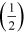past 2 or a quarter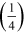to 6. The half and the quarter are parts of an hour; in other words they are fractions of a whole.

The number at the bottom of the fraction tells you how many parts the whole one has. So,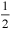has two parts in the whole one and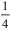has 4. The number at the top tells you how many of these parts there are. Hence,is 1 lot of 2 parts. You’ll be looking at this again in more detail in Week 3.

Since fractions are parts of a whole, just as decimals are, this means that you can relate fractions and decimals. Here you will only be dealing with fractions that are easily shown in a place value table.

So, say you needed to write out 2 and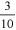(three-tenths) as a decimal. The whole part is 2 and the fractional part is. Remember in order to separate the whole number from the fraction you use a decimal point, with the part of the whole number to the right. So, you would write the 2 to the left of the decimal point and the fractional part to the right of the decimal point. Thus, it would be written as 2.3. If you were dealing withon its own, you would need show that there were no whole parts by showing a zero to the left of the decimal point.is therefore written as 0.3.

Now you’ve seen a few examples it’s your turn to have a try yourself in the next activity. You can use a place value table if it helps you.

## Activity 6 Fractions to decimals

Timing: Allow approximately 10 minutes

a) Rewrite each of the following fractions as a decimal number.

• i.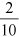### Comment

If the number does not have a whole number part, a zero is written in the units place. This makes the number easier to read (it’s easy to overlook the decimal point).

• i.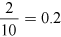• ii.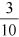• ii.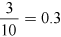• iii.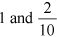• iii.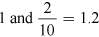• iv.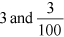• iv.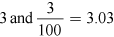b) Match the numbers below to the letter shown on the number line: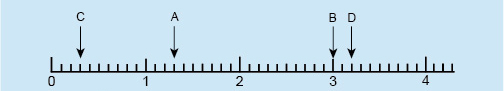Figure 13 Number line exercise

Using the following two lists, match each numbered item with the correct letter.

1. B

2. C

3. A

4. D

• a.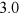• b.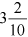• c.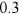• d.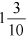• 1 = a
• 2 = c
• 3 = d
• 4 = b

Now, you’re going to turn your attention to rounding numbers, and making estimates. Understanding place value will help with this but you’ll also investigate the idea of decimal places when rounding.